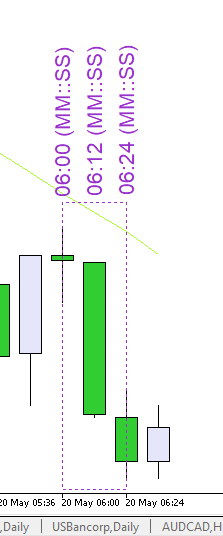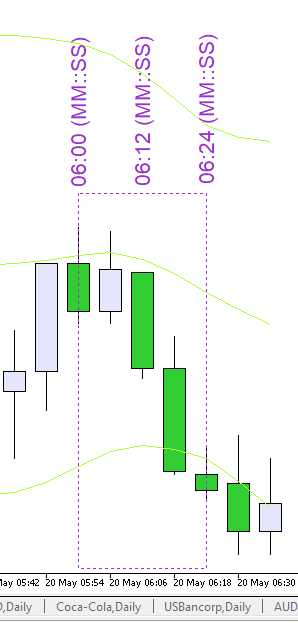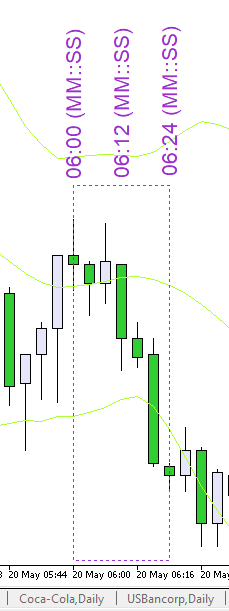# How to get candles from parent timeframe and lower contained timeframes using CopyRates() and populate them in array

I want to perform certain tasks on the open, high, low and close of the last but one (index of 1) M12 candle, and the M6 and M4 candle within it.

Since CopyRates() has this candle info I am using it to copy the candles.

How can I load exactly  one M12 candle (of index 1 when M12 candle closes) and two M6 candles and three M4 candles (M6 and M4 within M12) using the CopyRates? This seems to be getting me confused and I’m loading more than I need.

Secondly, how can I populate a new array with all these elements? I tried CopyArray but that only copies 1. I need to copy multiple arrays into one.

```MqlRates m_rates_m12[], m_rates_m6[], m_rates_m4[];
MqlRates combinedArrays[];

int OnInit()
{
//---
ArraySetAsSeries(m_rates_m12,true);
ArraySetAsSeries(m_rates_m6,true);
ArraySetAsSeries(m_rates_m4,true);

//---
return(INIT_SUCCEEDED);
}
//+------------------------------------------------------------------+
//| Expert deinitialization function                                 |
//+------------------------------------------------------------------+
void OnDeinit(const int reason)
{
//---
}
//+------------------------------------------------------------------+
//| Expert tick function                                             |
//+------------------------------------------------------------------+
void OnTick()
{

datetime curDispBarTime=0;
static datetime prevDispBarTime = 0;

curDispBarTime = iTime(_Symbol,PERIOD_M12,0);
bool isNewDispBar = curDispBarTime != prevDispBarTime;

if(isNewDispBar)  {

prevDispBarTime = curDispBarTime;

//--- PERIOD_M12
int start_pos=0; // start position
int count=3; // data count to copy
if(CopyRates(Symbol(),PERIOD_M12,start_pos,count,m_rates_m12)!=count)
return;
//--- PERIOD_M6
if(CopyRates(Symbol(),PERIOD_M6,m_rates_m12.time,m_rates_m12.time,m_rates_m6)!=3)
return;
//--- PERIOD_M4
if(CopyRates(Symbol(),PERIOD_M4,m_rates_m12.time,m_rates_m12.time,m_rates_m4)!=4)
return;

Print("m_rates_m12 first size is "+ArraySize(m_rates_m12));
Print("m_rates_m6 first size is "+ArraySize(m_rates_m6));
Print("m_rates_m4 first size is "+ArraySize(m_rates_m4));

}
}```Pic. 1. 'M12'Pic. 2. 'M6'Pic. 3. 'M4'

Pic. 1. 'M12'

Pic. 2. 'M6'

Pic. 3. 'M4'

Okay from the images lets say M12 at 06:00 just closed as in Pic.1. I want to get M12 06:00 candle, the two M6 candles within it and the three M4 candles within it using CopyRates(). That gives me a total of six elements from 3 different arrays.

Then I want to populate these 6 elements from the 3 arrays in a new array "CombinedArrays". This is what I've been trying to achieve and not sure how to fix from the code I provided.

BluePipsOnly :

Okay from the images lets say M12 at 06:00 just closed as in Pic.1. I want to get M12 06:00 candle, the two M6 candles within it and the three M4 candles within it using CopyRates(). That gives me a total of six elements from 3 different arrays.

Then I want to populate these 6 elements from the 3 arrays in a new array "CombinedArrays". This is what I've been trying to achieve and not sure how to fix from the code I provided.

From time to time to ...

From time to time to ...

BluePipsOnly :

Look carefully at the picture:Pic. 1. 'M12'

You should be interested in the 'M12' candle at 06:12 .

May I point you to another thread with a question and a solution which might also apply to your challenge.

https://www.mql5.com/en/forum/368332/page2#comment_22289555

Reason: